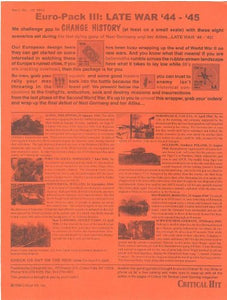Vendor
CRI Critical Hit
Regular price
Sold out
Sale price
\$31.99
Quantity must be 1 or more

• A

• S

• L

• A

• d

• v

• a

• n

• c

• e

• d

• S

• q

• u

• a

• d

• L

• e

• a

• d

• e

• r

• s

• c

• e

• n

• a

• r

• i

• o

• k

• i

• t

• w

• i

• t

• h

• 8

• s

• c

• e

• n

• a

• r

• i

• o

• s

• f

• r

• o

• m

• a

• c

• t

• i

• o

• n

• s

• i

• n

• t

• h

• e

• l

• a

• s

• t

• 2

• y

• e

• a

• r

• s

• o

• f

• t

• h

• e

• w

• a

• r

• i

• n

• E

• u

• r

• o

• p

• e

Set of 8 scenarios focusing on European actions on both fronts during thelast 2 years of the war. Created in Europe. '98